Question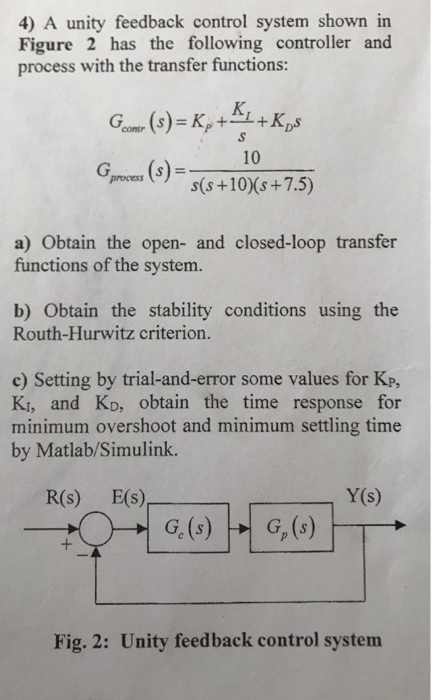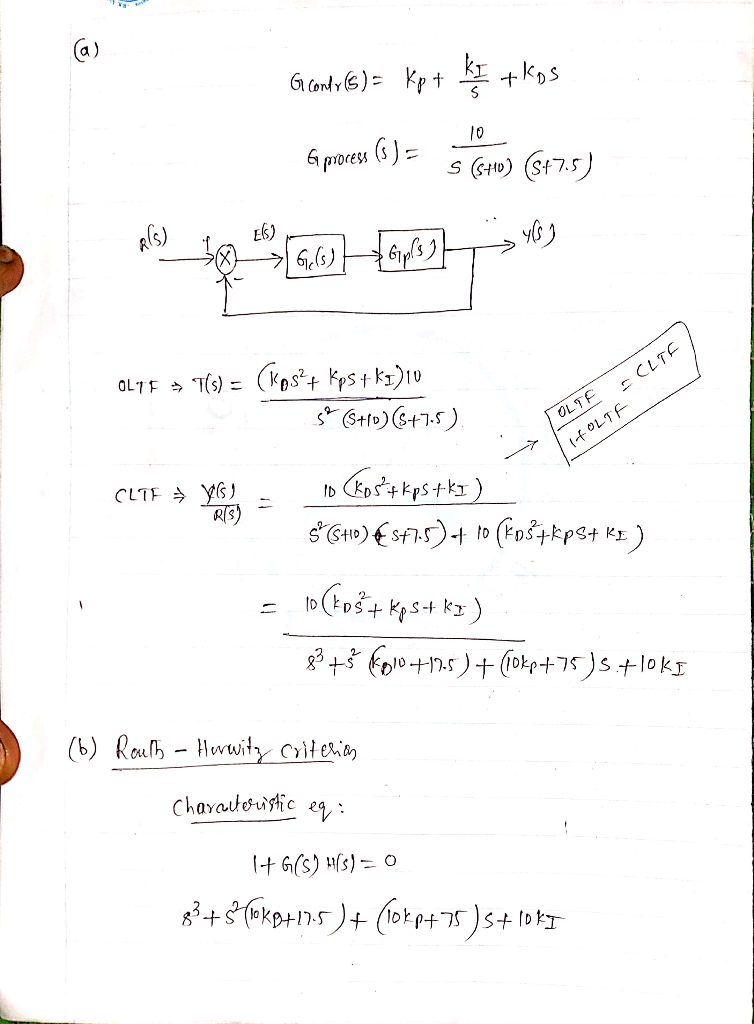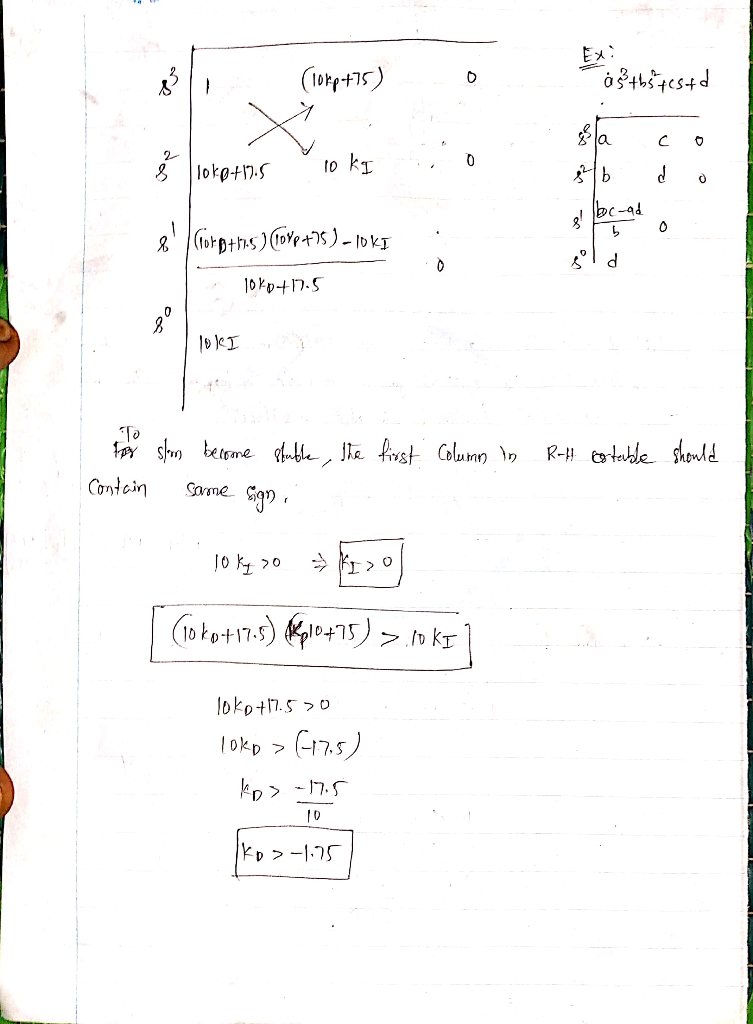#### Earn Coins

Coins can be redeemed for fabulous gifts.

Similar Homework Help Questions
• ### Consider a unity-feedback control system with a PI controller Gpr(s) and a plant G(s) in cascade. In particular, the plant transfer function is given as 2. G(s) = s+4, and the PI controller trans...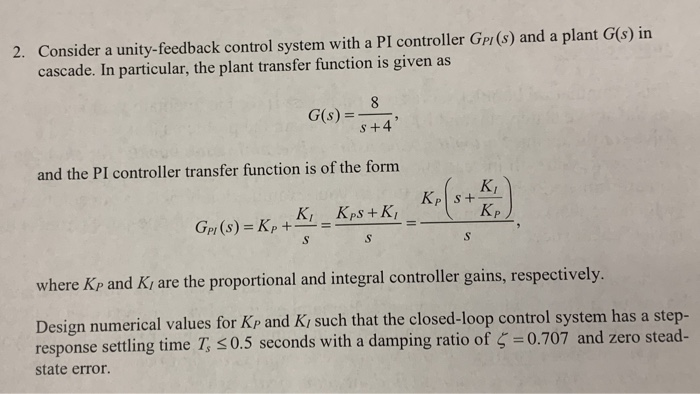Consider a unity-feedback control system with a PI controller Gpr(s) and a plant G(s) in cascade. In particular, the plant transfer function is given as 2. G(s) = s+4, and the PI controller transfer function is of the forrm KI p and Ki are the proportional and integral controller gains, respectively where K Design numerical values for Kp and Ki such that the closed-loop control system has a step- response settling time T, 0.5 seconds with a damping ratio of...

• ### A unity feedback control system has the following open loop transfer function, K L(s) .If K 10, evaluate the stability...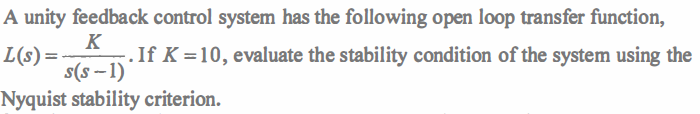A unity feedback control system has the following open loop transfer function, K L(s) .If K 10, evaluate the stability condition of the system using the s(s-1) Nyquist stability criterion. A unity feedback control system has the following open loop transfer function, K L(s) .If K 10, evaluate the stability condition of the system using the s(s-1) Nyquist stability criterion.

• ### A unity feedback system with the forward transfer function G)2)(s +5) is operating with a closed-...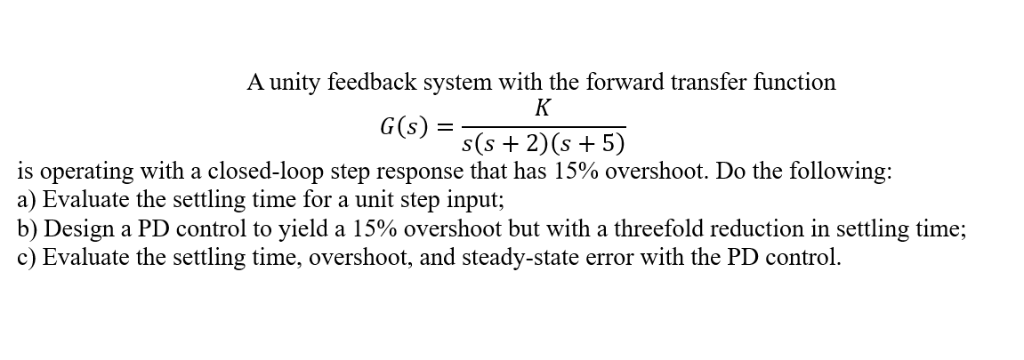A unity feedback system with the forward transfer function G)2)(s +5) is operating with a closed-loop step response that has 15% overshoot. Do the following: a) Evaluate the settling time for a unit step input; b) Design a PD control to yield a 15% overshoot but with a threefold reduction in settling time; c) Evaluate the settling time, overshoot, and steady-state error with the PD control. A unity feedback system with the forward transfer function G)2)(s +5) is operating with...

• ### TF= 0.033 / ( 1.6*10^(-7) *s +4.04*10^(-4) *s + 1.109*10^(-2) ) For unity feedback with P-Controller,...

TF= 0.033 / ( 1.6*10^(-7) *s +4.04*10^(-4) *s + 1.109*10^(-2) ) For unity feedback with P-Controller, solve the TF, find the value of K for a stable system using Root Locus and Routh-Hurwitz stability criterion.

• ### K and consider a PI s+4 A unity feedback system has an open loop transfer function G(s)  S+a controller Ge(s) S Sele...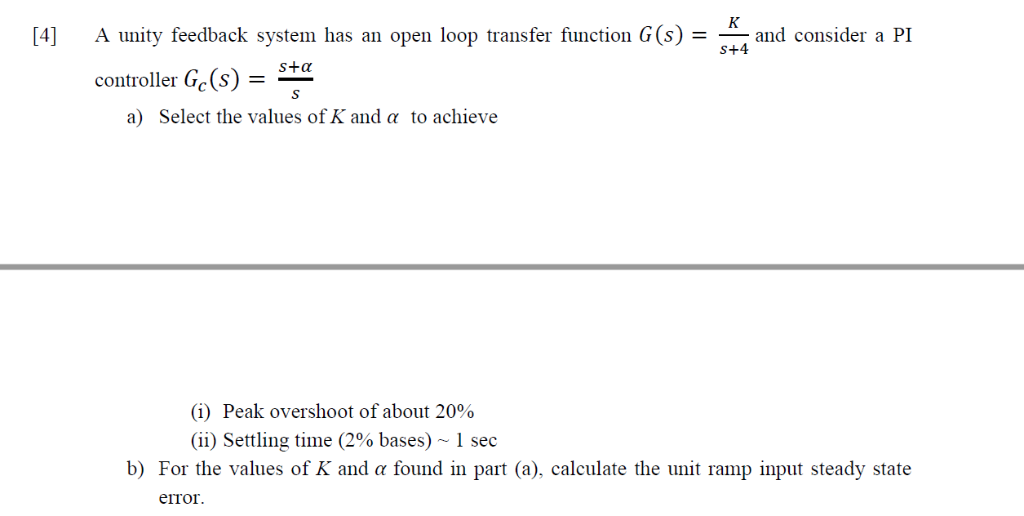K and consider a PI s+4 A unity feedback system has an open loop transfer function G(s)  S+a controller Ge(s) S Select the values of K and a to achieve a) (i) Peak overshoot of about 20% (ii) Settling time (2% bases) ~ 1 sec b) For the values of K and a found in part (a), calculate the unit ramp input steady state error K and consider a PI s+4 A unity feedback system has an open loop...

• ### 6 and controller C(s), as shown in the Consider a unity-feedback control system with plant G(s)-...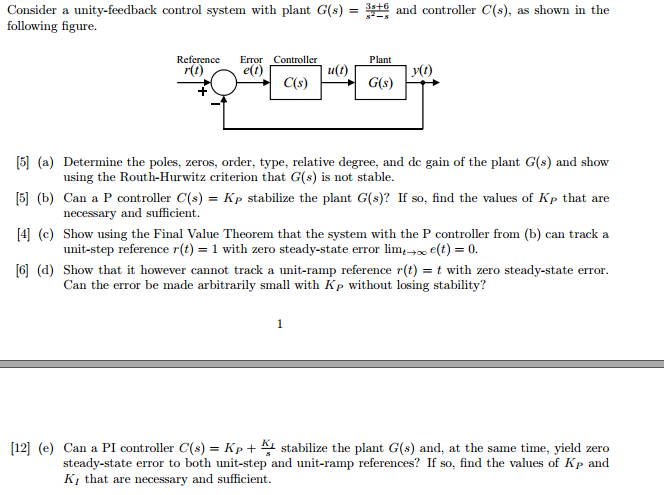6 and controller C(s), as shown in the Consider a unity-feedback control system with plant G(s)- following figure. Reference Error Controller Plant r(t) e(t) u(t) y(t) C(s) G(s)  (a) Determine the poles, zeros, order, type, relative degree, and de gain of the plant G(s) and show  (b) Can a P controller C(s)Kp stabilize the plant G(s)? If so, find the values of Kp that are  (c) Show using the Final Value Theorem that the system with the...

• ### Question 6 The open-loop transfer function G(s) of a control system is given as G(8)- s(s+2)(s +5...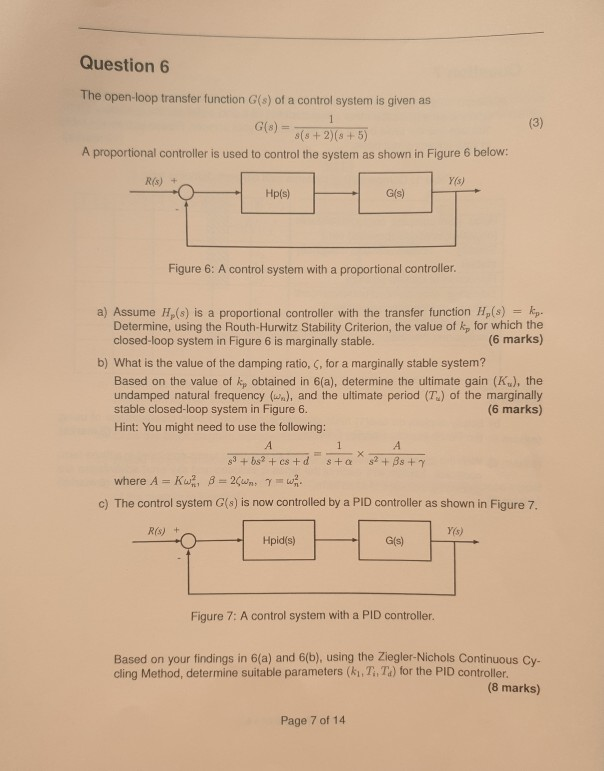Question 6 The open-loop transfer function G(s) of a control system is given as G(8)- s(s+2)(s +5) A proportional controller is used to control the system as shown in Figure 6 below: Y(s) R(s) + G(s) Figure 6: A control system with a proportional controller a) Assume Hp(s) is a proportional controller with the transfer function H,(s) kp. Determine, using the Routh-Hurwitz Stability Criterion, the value of kp for which the closed-loop system in Figure 6 is marginally stable. (6...

• ### TF= 0.033 / ( 1.6*10^(-7) *s +4.04*10^(-4) *s + 1.109*10^(-2) ) For unity feedback with P-Controller, solve the TF, find...

TF= 0.033 / ( 1.6*10^(-7) *s +4.04*10^(-4) *s + 1.109*10^(-2) ) For unity feedback with P-Controller, solve the TF, find the value of K for a stable system using Root Locus and Routh-Hurwitz stability criterion.

• ### A unity feedback closed loop control system is displayed in Figure 4. (a) Assume that the control...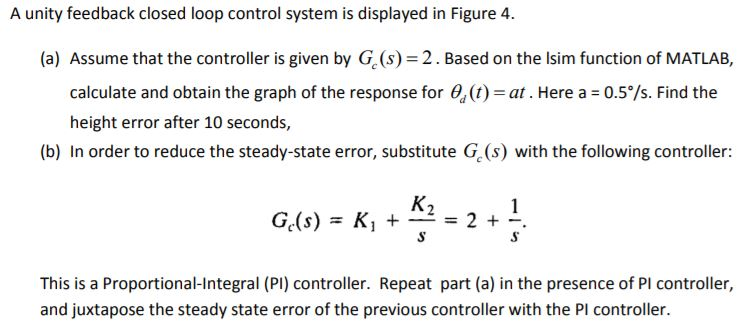Please solve as a MATLAB code. A unity feedback closed loop control system is displayed in Figure 4. (a) Assume that the controller is given by G (s) 2. Based on the lsim function of MATLAB, calculate and obtain the graph of the response for (t) at. Here a 0.5°/s. Find the height error after 10 seconds, (b) In order to reduce the steady-state error, substitute G (s) with the following controller This is a Proportional-Integral (PI) controller. Repeat part...

• ### 1. Consider the usual unity-feedback closed-loop control system with a proportional-gain controll...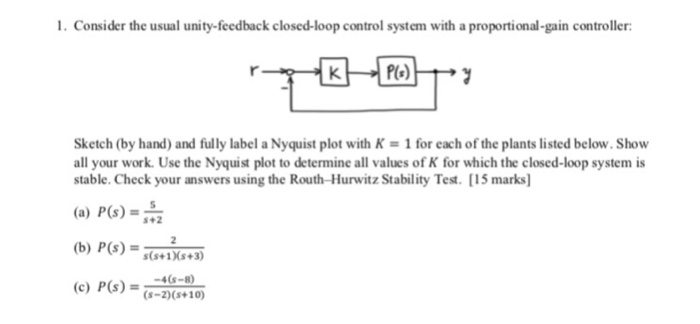1. Consider the usual unity-feedback closed-loop control system with a proportional-gain controller Sketch (by hand) and fully label a Nyquist plot with K-1 for each of the plants listed below.Show all your work. Use the Nyquist plot to determine all values of K for which the closed-loop system is stable. Check your answers using the Routh-Hurwitz Stability Test. [15 marks] (a) P(s)-2 (b) P(s)-1s3 (c) P(s) -4-8 s+2 (s-2) (s+10) 1. Consider the usual unity-feedback closed-loop control system with a...